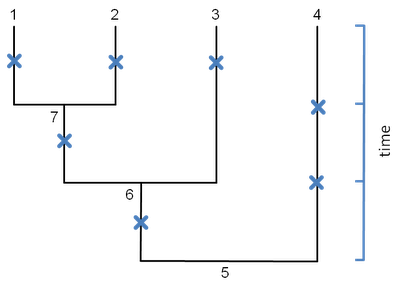## Tuesday, May 3, 2011

### Bug fix and update to min.split()

I just fixed and updated my function min.split(). This function can be used to analyze the posterior sample of rate shift positions generated by evol.rate.mcmc() (described here: 1,2,3,4,5,6; and in an upcoming paper).

The bug caused the distance between two points on the same edge to be miscalculated. Oops! This should have been the easy part! Any users of evol.rate.mcmc() who have used min.split() should definitely update to v0.5 (here).

The update now allows the user to find the split in a sample that minimizes the summed or the summed squared distances to all the other points in the sample. It can also (optionally) print the distance matrix among splits.

The way the function works is as follows. Take the following set of points on the tree below:which we represent in a list as follows:

> nodelist<-matrix(c(1,2,7,6,3,4,4,0.5,0.5,0.5,0.5,1.5,1,2), 7,2,dimnames=list(NULL,c("node","bp")))
> nodelist
node bp
[1,] 1 0.5
[2,] 2 0.5
[3,] 7 0.5
[4,] 6 0.5
[5,] 3 1.5
[6,] 4 1.0
[7,] 4 2.0

The syntax here is simple. "node" is the node number in \$edge; and "bp" is just the distance along the preceding edge from the node below (not from the "node").

We can then run min.split() as follows:

> source("evol.rate.mcmc.R")
> min.split(tree,nodelist,printD=TRUE)
[,1] [,2] [,3] [,4] [,5] [,6] [,7]
[1,] 0.0 1.0 1.0 2.0 3.0 3.5 4.5
[2,] 1.0 0.0 1.0 2.0 3.0 3.5 4.5
[3,] 1.0 1.0 0.0 1.0 2.0 2.5 3.5
[4,] 2.0 2.0 1.0 0.0 2.0 1.5 2.5
[5,] 3.0 3.0 2.0 2.0 0.0 3.5 4.5
[6,] 3.5 3.5 2.5 1.5 3.5 0.0 1.0
[7,] 4.5 4.5 3.5 2.5 4.5 1.0 0.0
\$node
7
\$bp
0.5

Which returns the minimum split (in this case node=7,bp=0.5), and, with printD=TRUE, prints to screen the distance matrix among points in the row order of nodelist.

To compute the summed squared distances instead of the summed distances, we just need to do the following:

> min.split(tree,nodelist,method="sumsq")
\$node
6
\$bp
0.5

and that's it!

1.2.